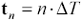# Discrete Time Mapping

The FFT requires that you identify the mapping between the continuous-time world and the discrete-time world. This is done with two parameters, D T and N .

The parameter D T defines the time interval between successive samples of the waveform to be transformed. The samples are evenly spaced . The spacing D T must be small enough to fairly represent the complete signal waveform without loss of significant information. This implies that on a scale of time commensurate with one sample, the signal waveform had better look smooth, not changing very much between samples.

For example, if you are working with a waveform having a 10% to 90% percent rise and fall time of t 10-90 , then D T should be set anywhere from 4 to 100 times smaller than t 10-90 . Gaussian-shaped rising and falling edges require only a 4x oversampling . Parabolic edges (as created by some IBIS simulators) require about 10x. Linear-ramp edges require much higher sampling rates. Plain square edges, no matter how fast you sample them, create special sampling problems that require fantastic sampling speeds.

The requirement for a small D T is roughly equivalent to a requirement that the sampling rate 1/ D T exceed twice the highest frequency contained in the sampled waveform. Precisely defining the highest frequency can be somewhat problematic . The general rule is that if the spectral content of your signal is down to “60 dB below the meat of the signal spectrum at frequency f 1 , and if the spectrum above f 1 plummets at a rate of at least “40 dB from that point onward, then a sampling rate of 2 f 1 should provide a discrete-time conversion accuracy on the order of one part in one thousand.

One simple test of sampling efficacy is to halve the sampling interval and look for any observable change in the computed spectrum. A noticeable change indicates the original sampling rate was inadequate. Factors that dictate very high oversampling rates include outrageous requirements for time-domain accuracy, investigation of high-frequency EMC phenomena occurring at large multiples of the signal bandwidth, and inadequate low-pass filtering in the system you are trying to model. If you see results that change markedly with a small shift in the sampling phase, that's another sign of sample-rate inadequacy.

Another test of sampling sufficiency is made by observing the magnitude of your FFT output. If the magnitude as a function of frequency is not quickly diminishing toward zero at 1/2 the sampling rate, then your output will most certainly suffer noticeable amounts of aliasing (see Section 4.7, "Effect of Inadequate Sampling Rate").

When working with simulated signals that have linear ramps or square edges, I always include in the simulation a model of a scope probe with limited bandwidth. The scope probe model limits the spectral content of the observed signals (just as in real life), so you can safely use a finite sampling rate to process the simulated waveforms.

The parameter N defines the length of the time-domain sample vector. In conjunction with D T it defines the total window of time ( N · D T ) available within which the FFT must do its work. Always provide an N large enough to allow your simulated system to come to a steady-state condition before the end of the FFT time window. This condition comes about because FFT time, unlike time in the real world, wraps back around onto itself in a loop. Any residual tail in your waveform that " falls off the end of time" doesn't really fall off, but wraps back onto the beginning of time, superimposing onto and distorting your view of the initial circuit conditions.

In rare cases the wrapping helps. For example, when analyzing a clock waveform, if you establish the parameters such that N · D T equals a precise multiple of the clock period, then the tail of the last clock cell overlaps with the beginning of the first period in a manner reminiscent of how the circuit would actually work in continuous time. Most often, though, the wrapping is a bothersome artifact of the FFT approximation and must be dealt with by providing sufficient additional time at the end of the FFT time window to allow your system to fully stabilize to within a negligible tolerance. When working with channels subject to the skin-effect the full stabilization time can be quite long.

When you plot discrete-time waveforms, it is handy to precompute a horizontal axis vector t . The horizontal time axis t n associated with each point x n in the sampled time-domain vector depends on the index n and the step interval D T .

Equation 4.5POINTS TO REMEMBER

• The FFT requires two parameters: a sample interval D T and a sample vector length N .
• The spacing D T must be small enough to fairly represent the complete signal waveform without loss of significant information.
• Always provide an N large enough to allow your simulated system to come to a steady-state condition before the end of the FFT time window.High-Speed Signal Propagation[c] Advanced Black Magic
ISBN: 013084408X
EAN: N/A
Year: 2005
Pages: 163